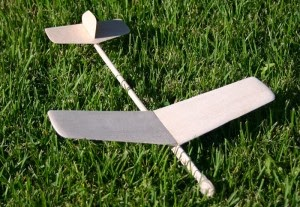## Wednesday, February 26, 2014

### Using Online Calculator to Compute Lift

Most likely one of the first formulas one would see when studying aerodynamics is the formula for lift.  It can be seen re-written from various sources but it is the same formula.  I have been interested in applying the formula to model aviation but a couple of units of measure in the formula are not what you would normally work with in model aviation. The surface area units (square feet) is what would be easier to use with full-scale airplanes and velocity in feet per second is not what most of us think of.  To make this all easier I ran across lift calculator program on the Internet that makes it easy to work with whatever units your data is in.Airplane in Level Flight Flies With Slight  Positive Angle of Attack

Lift Formula

### Lift = Coefficient of Lift x .5 x Density of Air x Velocity² x Surface Area

The variables in the equation need further explanation.

Coefficient of Lift (cl)  is created to take several factors into account but the most dominant would be the angle of attack of the wing. Other factors would be the shape of the airfoil including the thickness. Coefficient is a word that has a different meaning in math but in physics it is a quantitative expression of some type of property; coefficient of friction is a very common coefficient.

Density of Air is mass per unit volume of Earth's atmosphere and can be found in tables. Factors to this variable are altitude, humidity, and temperature. This is why jet aircraft need to build up more speed to take off on hot days and from high altitude runways.

Velocity
is the speed that the air is moving over the wing horizontally from the front of the airfoil. It is labeled velocity because it is a vector quantity, the air is moving from a specified direction. In the formula this is specified in feet per second but miles per hour is what most people in the US think of. Note velocity is squared, this means it is the dominant variable in the equation and the reason that the amount of lift increases substantially as velocity increases.

Surface Area is given in the formula in square feet which is great for full-scale aircraft but square inches or square centimeters would be easier to relate to with model aircraft.My Hand Launch Glider Converted to Catapult Launch

Online Calculator Website:

With the calculator website I wanted to give it a try but I did not have any data currently but thought of an article on the AMA Glider website Kurt Krempetz entitled, “Catapult Glide Time Prediction Theories.” This isn’t exactly what I wanted because unless a glider is in lift, the amount of lift is slightly less than the weight of the glider because it is going down the glide slope.  From the article I was able to retrieve weight of the glider, wing surface area, velocity, density of air, and CL (coefficient of lift).

Coefficient of Lift  - .34
Weight of Glider – 5.5 grams
Wing Surface Area – 28 square inches
Velocity – 12.86 feet per second
Density of Air - .071 pounds per cubic footData Plugged into Online Calculator

Plugging all of this into the calculator gives 5.47 grams of force which is slightly less than the 5.5 grams weight of the glider which seems completely reasonable. By the way, 12.86 feet per second is about 8.77 miles per hour.

I really need to think more about how I might use this tool, to me it is fascinating to see how math works out with real world applications.

Bill Kuhl
http://www.ideas-inspire.com